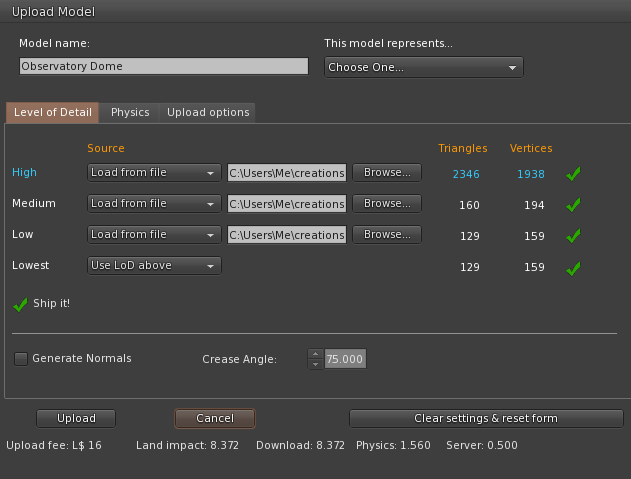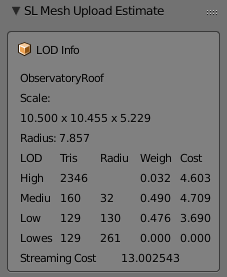## The truth about mesh streaming

Ever wondered why a mesh with the same number of triangles could give different LI? Or how the impact of each LOD model is assessed? Stick with me today and hopefully, I'll show you.

Today's post is the fifth in the series of meanderings through Blender Addons. Yesterday, we left things in an OK state. My AddOn is reflecting the correct triangle counts for the models (and correctly associating the models with the LOD they represent).

Today we will look at the Mesh Streaming Cost algorithm and have a go at converting that to python.
This is an unashamedly technical blog. I will try to explain some aspects as I go through but the nature of the topic demands some technical detail, quite a lot of it.

I am going to work from the latest Firestorm Viewer source, and a couple of somewhat outdated wiki resources. The wiki resources themselves should be good enough, but the problem with them is that you can never be sure if things have been tweaked since. Ultimately though we have a real world comparison, our estimates should match (or be close to) the Viewer upload, we will test this at the very end.

A good place to start is the Mesh Streaming Cost wiki page as with many wiki documents it is out of date and not entirely correct. However, we can use it as a starting place. The concept section explains the thought behind this. The equation part is where we will start.
1. Compute the distance at which each LOD is displayed
2. Compute the area in which each LOD is relevant
4. Scale relative weights of each LOD based on what percentage of the region each LOD covers.
5. Compute cost based on relevant range and bytes in LOD
It goes on to tell us what the LOD transition distances are, details we covered in the post yesterday.

Using these we can write another helper function
```def getLODRadii(object):
max_distance = 512.0
dlowest = min(radius / 0.03, max_distance)
dlow = min(radius / 0.06, max_distance)
dmid = min(radius / 0.24, max_distance)
```

This function takes an object and using our previously written radius function and applying the knowledge above, returns a list of values. The radius itself, the High to Mid transition distance, The Mid to low transition and finally the Low to Lowest.

We use a constant max distance of 512 as it matches that used in the code example and the current live code. Quite why it should be 512 (2 regions) is unclear to me.

So now we should be able to add a new column to our display and show the LOD change radii

Step 2 is to compute the area for each LOD. Now that we have the Radius that is a simple task.

```def area_of_circle(r):
return math.pi * r * r
```

The function above returns the area for a given radius.

The next step is "Adjusting for missing LODs", we'll take this into account when we display things. But in terms of the algorithm, if a given LOD is missing then the next highest available LOD is used.

We can now progress to the "Computing Cost" section. This section gives use the following formula.

```    Streaming Cost =
(   (lowest_area / total_area) * bytes_in_lowest
+ (low_area    / total_area) * bytes_in_low
+ (mid_area    / total_area) * bytes_in_mid
+ (high_area   / total_area) * bytes_in_high   ) * cost_scalar```
The first part is a ratio, a weighting applied to the LOD based upon the visibility radii.
The second part is more confusing on its own, "bytes_in_LOD" where did that come from?

The answer lies in the note just below the pseudo code.
In the details of the implementation, the `cost_scalar` is based on a target triangle budget, and efforts are made to convert `bytes_in_foo` to an estimated triangle count.
So what does that mean exactly? The answer lies in the C++ code below it and, in particular:
`F32 bytes_per_triangle = (F32) gSavedSettings.getU32("MeshBytesPerTriangle");`
This is a setting stored in the viewer that approximates how many bytes are in a triangle for the purpose of converting "bytes" to triangles. Looking at the current live viewers, we find that the setting has a value of 16.

This value is then used to convert a bytes_LOD value to a triangles_LOD value.
```    F32 triangles_high   = llmax((F32) bytes_high-METADATA_DISCOUNT, MINIMUM_SIZE
/bytes_per_triangle;```
This deducts a METADATA_DISCOUNT constant to remove the "overhead" in each mesh LOD to leave only the real triangle data. The remaining bytes are divided by our bytes_per_triangle to get the number of triangles. This raises the question of whether 16 is the right "estimate" Indeed, why is it an estimate at all? In Blender we won't be estimating, we know how many triangles we have. However, it will turn out that the page is missing one vital piece of information that explains all of this...However, we will come back to this once we have worked out the rest.

Looking in more detail at the implementation we find that lowest area and the related "areas" are not quite what they seem.
In the C++ implementation, we observe that high_area is indeed the area of the circle defined by the roll off point from High to Medium LOD,
`F32 high_area   = llmin(F_PI*dmid*dmid, max_area);`
but we discover that mid_area is the area of the medium range only, excluding the high_area. The area of the Ring in which the Medium LOD is visible.The same applies to the others.

Putting this all together in python we get the following:-

```def getWeights(object):

MaxArea = bpy.context.scene.sl_lod.MaxArea
MinArea = bpy.context.scene.sl_lod.MinArea

highArea = clamp(area_of_circle(LODSwitchMed), MinArea, MaxArea)
midArea = clamp(area_of_circle(LODSwitchLow), MinArea, MaxArea)
lowArea = clamp(area_of_circle(LODSwitchLowest), MinArea, MaxArea)
lowestArea = MaxArea

lowestArea -= lowArea
lowArea -= midArea
midArea -= highArea

highArea = clamp(highArea, MinArea, MaxArea)
midArea = clamp(midArea, MinArea, MaxArea)
lowArea = clamp(lowArea, MinArea, MaxArea)
lowestArea = clamp(lowestArea, MinArea, MaxArea)

totalArea = highArea + midArea + lowArea + lowestArea

highAreaRatio = highArea / totalArea
midAreaRatio = midArea / totalArea
lowAreaRatio = lowArea / totalArea
lowestAreaRatio = lowestArea / totalArea
return (highAreaRatio, midAreaRatio, lowAreaRatio, lowestAreaRatio)
```

This should give us the weighting of each LOD in the current models at the current scale. So let's add this to our display.

Here we can see that our Medium and Low LODs are carrying a lot of the LI impact and thus if we want to manage the LI we need to pay a lot of attention to these. The more observant will note that the Lowest is effectively 0, and yet we are telling it to use the LOD from the LOW, this makes no sense at first glance, it should be very expensive. The explanation is in the radius column. Lowest does not become active until 261m, which is outside of the 256m maximum  (see maxArea in the code above), this means that the Lowest is clamped to a radius of 256, which matches the radius of the Low and thus results in 0 weight.

With all this in place, we are finally able to have a first run at calculating the streaming cost.
Once again we refer to the C++ implementation for guidance.
```    F32 weighted_avg = triangles_high*high_area +
triangles_mid*mid_area +
triangles_low*low_area +
triangles_lowest*lowest_area;

return weighted_avg/gSavedSettings.getU32("MeshTriangleBudget")*15000.f;```
In our python translation this becomes:

```        weightedAverage =   hi_tris*highAreaRatio + mid_tris*midAreaRatio + low_tris*lowAreaRatio + lowest_tris*lowestAreaRatio
streamingCost = weightedAverage/context.scene.sl_lod.MeshTriangleBudget*15000
```

I am not a fan of the magic numbers used here (MeshTriangleBudget is in fact another viewer setting and has a value of 250000, the 15000 however is a simple hard coded constant so we have little choice but to replicate it.

For our final reveal for tonight then let's see how out LI calculation has performed.Oh dear...
Well, I guess it had all been too easy so far.
The Firestorm upload has calculated that this object will have a streaming impact of 8LI
Our determination has calculated 13LI. That is a considerable difference, what could possibly have gone wrong?

The answer was hinted at previously; it is to do with the estimate, the bytes_LOD values and what they actually are. The problem lies in the fact that your mesh is not sent back and forth unaltered from the DaE file that you upload. In fact, it is uploaded in an internal format that compresses each LOD model. The bytes_LOD values represent the compressed size of the actual mesh that will be streamed, the estimated bytes_per_triangle of 16 is, it would seem greatly underestimating the compression level.
In my next blog, I will examine the internal format in more detail. We'll explain why the estimated bytes per triangle is wrong, and we will start to work out how we can make this work.

Until then, thank you for reading this blog. Please share or +1 if you have found it useful, or if you think that your friends might.

Love
Beq
x